# 数据挖掘入门系列教程（四）之基于scikit-lean实现决策树

2019/04/10 10:10

## 数据挖掘入门系列教程（四）之基于scikit-lean决策树处理Iris### 加载数据集

``````from sklearn.datasets import load_iris
data = dataset.data
target = dataset.target``````

``````import numpy as np
import pandas as pd
data = pd.DataFrame(data,columns=["sepal_length","sepal_width","petal_length","petal_width"])
data["class"] = target``````

data的数据如下所示：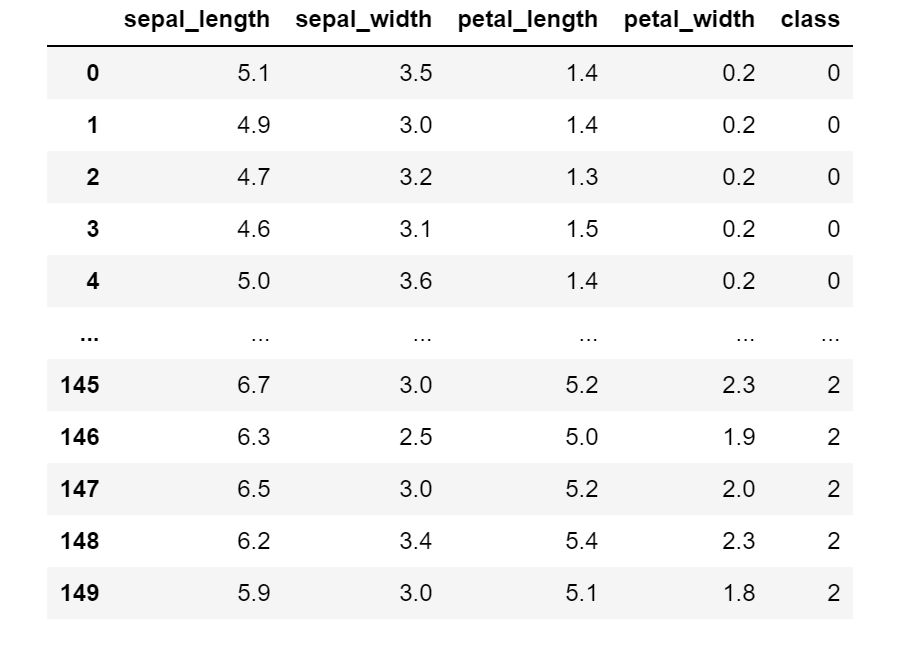class代表类别。其他的就是Iris的属性了。

### 数据特征

• matplotlib
• seaborn

``````import matplotlib.pyplot as plt
import seaborn as sb
# data.dropna()去除里面的none元素
sb.pairplot(data.dropna(),hue="class")``````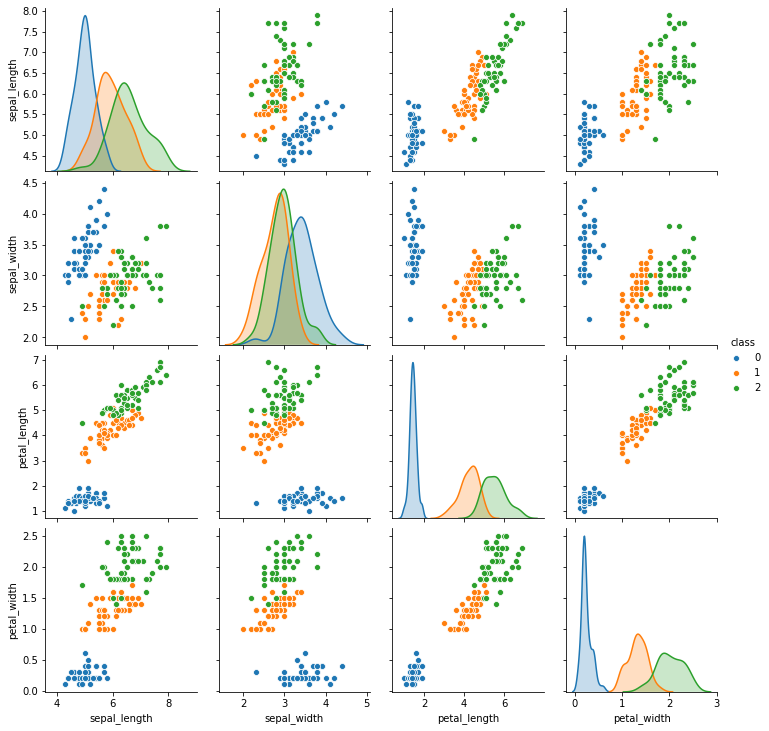``````plt.figure(figsize=(20, 20))
for column_index, column in enumerate(data.columns):
if column == 'class':
continue
plt.subplot(2, 2, column_index + 1)
sb.violinplot(x='class', y=column, data=data)``````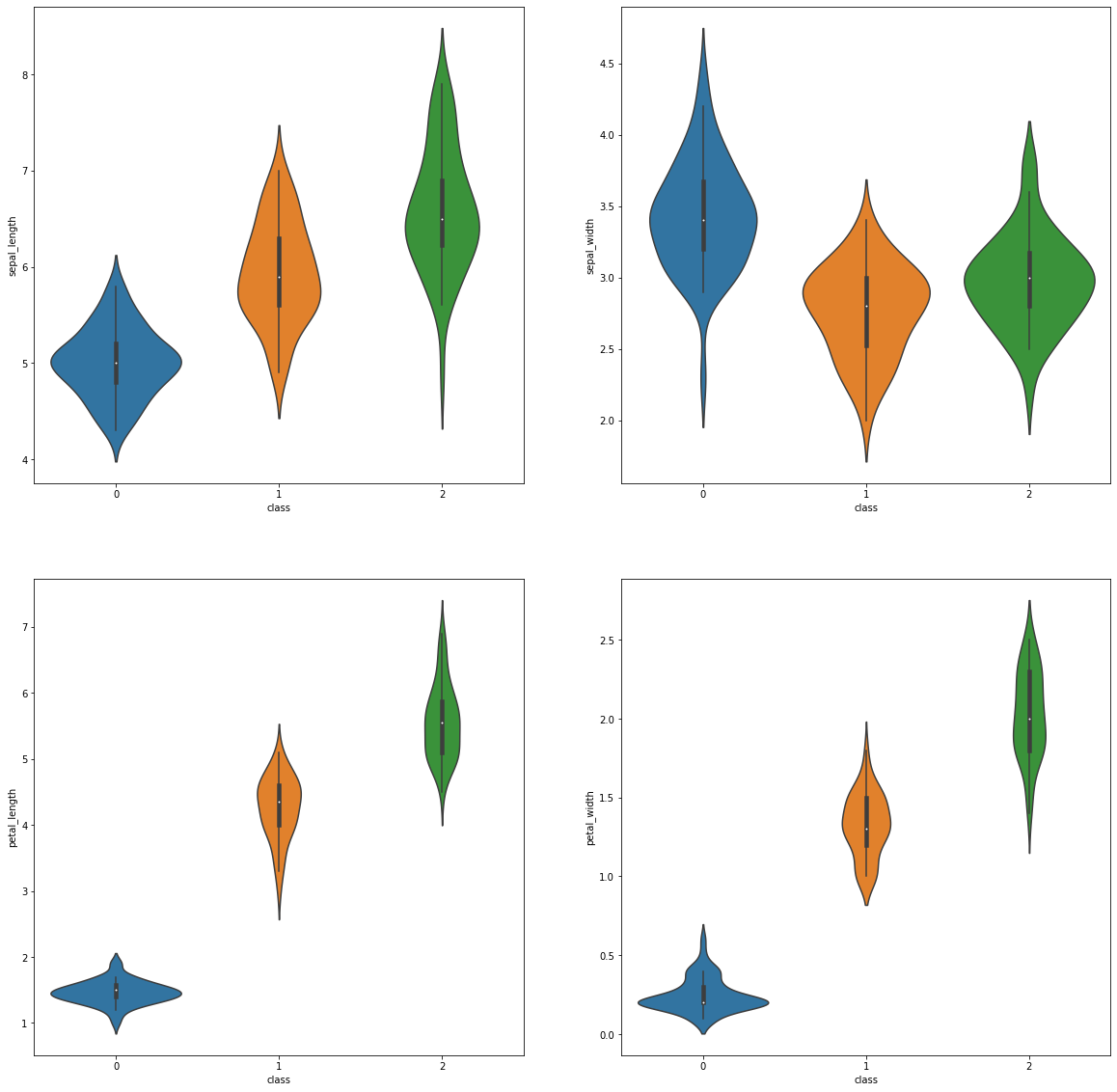### 训练

``````from sklearn.model_selection import train_test_split

input_data = data[["sepal_length","sepal_width","petal_length","petal_width"]]
input_class =  data["class"]

train_data,test_data,train_class,test_class = train_test_split(input_data,input_class,random_state = 14)``````

then，让我们来开始进行训练吧，在scikit-learn中实现了决策树，和前面的K近邻算法一样我们直接引用就行，调用fit（训练）和predict（预测）函数。使用如下所示：

``````from sklearn.tree import DecisionTreeClassifier

decision_tree = DecisionTreeClassifier(random_state=14)
decision_tree.fit(train_data,train_class)
predict_class = decision_tree.predict(test_data)
predict_score = np.mean(predict_class == test_class)
print("预测的准确度为{}".format(predict_score))``````

DecisionTreeClassifier其他的参数在后面说，这里主要说一下`random_state`参数。为什么决策树还需要random_state这个参数，以下知乎上面的两位博主的说法。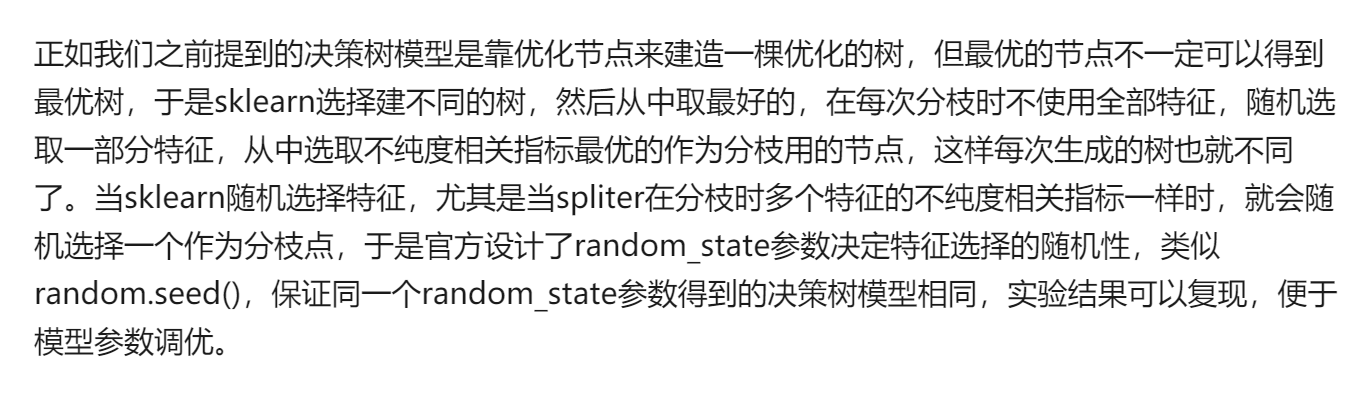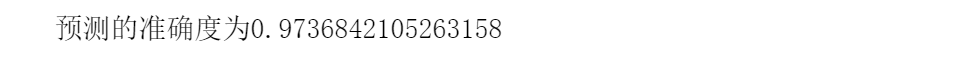``````# criterion  gini(默认)/tropy：这里对应的就是之前的熵增益和Gini系数

# splitter  best(默认)/random 每个结点选择的拆分策略

# max_depth  树的最大深度。

# min_samples_split int类型或者float(默认2) 如果某节点的样本数少于min_samples_split，则不会进行拆分了。浮点值表示分数，代表所占比例

# min_samples_leaf 默认=1 这个值限制了叶子节点最少的样本数，如果某叶子节点数目小于样本数，则会和兄弟节点一起被剪枝。

# min_weight_fraction_leaf float（默认0.0） 这个值限制了叶子节点所有样本权重，如果小于这个值，则会和兄弟节点一起被剪枝。一般来说，如果我们有较多样本有缺失值，或者分类树样本的分布类别偏差很大，就会引入样本权重，这时我们就要注意这个值了。

# max_features int, float or {“auto”, “sqrt”, “log2”}（默认0.0）

# max_leaf_nodes 通过限制最大叶子节点数，可以防止过拟合，默认是"None”，即不限制最大的叶子节点数。如果加了限制，算法会建立在最大叶子节点数内最优的决策树。

# class_weight dict/balanced 指定样本各类别的的权重，主要是为了防止训练集某些类别的样本过多导致训练的决策树过于偏向这些类别。这里可以自己指定各个样本的权重。“balanced”，则算法会自己计算权重，样本量少的类别所对应的样本权重会高。

# min_impurity_split 这个值限制了决策树的增长，如果某节点的不纯度(基尼系数，信息增益，均方差，绝对差)小于这个阈值则该节点不再生成子节点。即为叶子节点 。``````

``````from sklearn.tree import export_graphviz
with open("tree.dot",'w') as f:
export_graphviz(decision_tree, feature_names =['sepal_length', 'sepal_width', 'petal_length', 'petal_width'], out_file = f)``````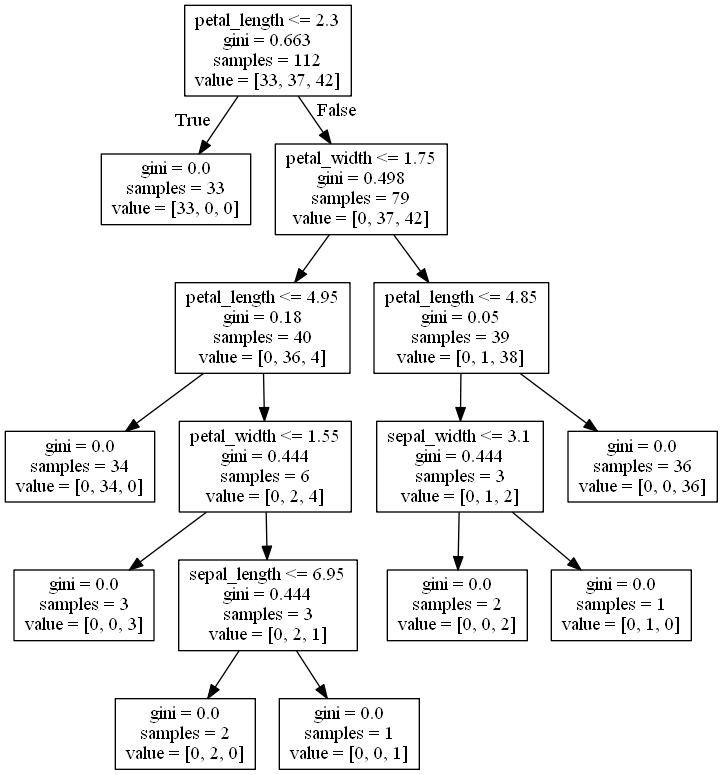``````from sklearn.model_selection import cross_val_score
decision_tree = DecisionTreeClassifier()
scores = cross_val_score(decision_tree,input_data,input_class,scoring='accuracy')
print("交叉验证结果: {0:.2f}%".format(np.mean(scores) * 100))``````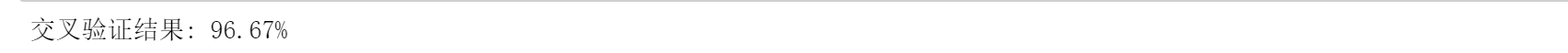### 随机森林

``````from  sklearn.ensemble import RandomForestClassifier
rft = RandomForestClassifier(n_estimators=20,random_state=14)
rft.fit(train_data,train_class)
predict_class = rft.predict(test_data)
predict_score = np.mean(predict_class == test_class)
print("随机森林预测的准确度为{}".format(predict_score))````````````scores = cross_val_score(rft,input_data,input_class,scoring='accuracy')
print("Accuracy: {0:.2f}%".format(np.mean(scores) * 100))``````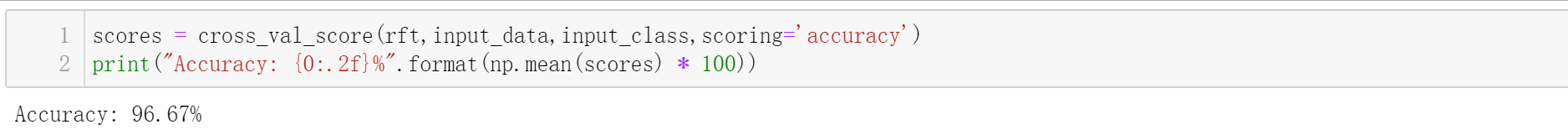emm，好像和上面的结果一样，因为这个数据集很小，可能会有这种情况。

### 调参工程师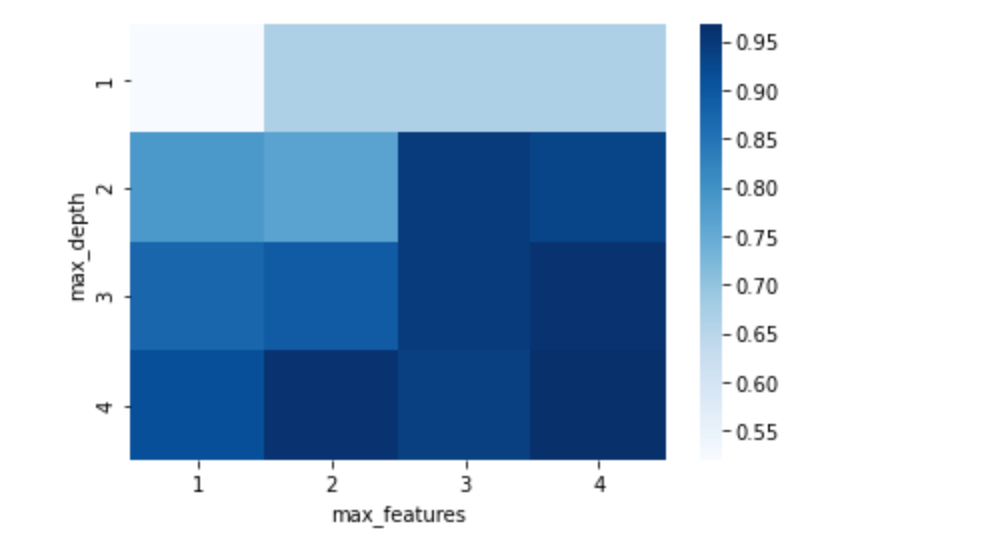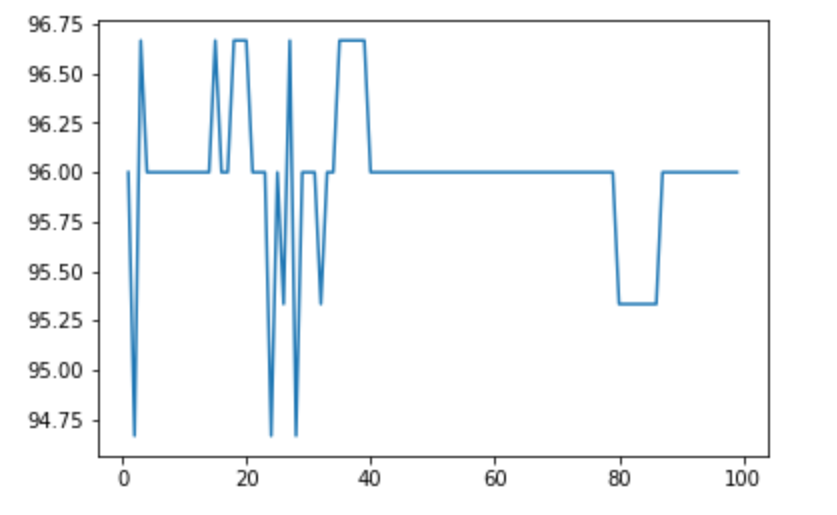0
0 收藏

### 作者的其它热门文章0 评论
0 收藏
0